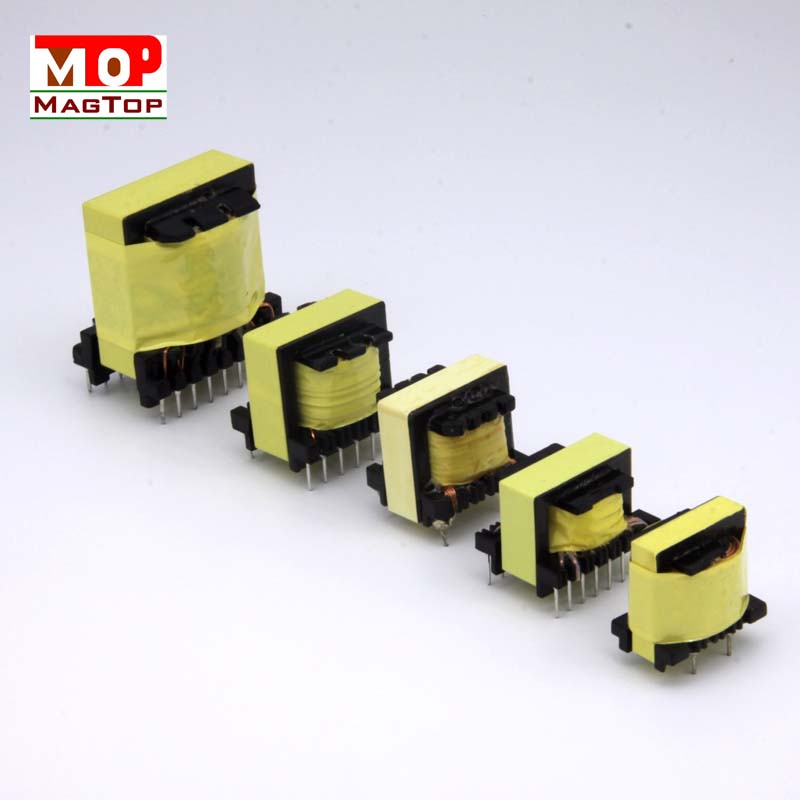# What are the main parameters of the transformer

##### author: MagTop
14/02/2023The main parameters of current transformer are voltage ratio, frequency characteristic, rated power and efficiency.
(1) Voltage ratio n The voltage ratio n of the transformer is related to the number of turns of the primary winding and the voltage as follows :n=V1N2=N1/N2, where N1 is variable voltage
N2 is the secondary winding, V1 is the voltage at both ends of the primary winding and V2 is the voltage at both ends of the primary winding.
Boost the voltage of the transformer
If the ratio n is less than 1, the voltage ratio n of the step-down transformer is greater than 1, and the voltage ratio of the isolation transformer is equal to 1.
(II) Rated power P This parameter is generally used for power transformers.
It refers to the power transformer in the specified working frequency and voltage, can work for a long time without exceeding
The output power over the specified temperature.
The rated power of the transformer is related to the core cross-sectional area and enamelled wire diameter.
Transformer core cross-sectional area is large, enamelled wire straight
Large diameter, its output power is also large.
3) Frequency characteristics Frequency characteristics refer to the transformer has a certain operating frequency range, and transformers with different operating frequency ranges are generally not used interchangeably.
Due to the
When the high power switching transformer works outside its frequency range, the temperature will rise or the transformer will not work normally.
Efficiency refers to the ratio of transformer output power to input power at rated load.
The value is proportional to the output power of the transformer, that is, the transformer
The greater the output power of the transformer, the higher the efficiency: the smaller the output power of the transformer, the lower the efficiency.
The efficiency value of transformer is generally between 60% and 100%